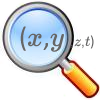# Resources tagged with: Trigonometric functions and graphs

Filter by: Content type:
Age range:
Challenge level:

There are 14 NRICH Mathematical resources connected to Trigonometric functions and graphs, you may find related items under Coordinates, Functions and Graphs.

Broad Topics > Coordinates, Functions and Graphs > Trigonometric functions and graphs### Tangled Trig Graphs

##### Age 16 to 18Challenge Level

Can you work out the equations of the trig graphs I used to make my pattern?### Taking Trigonometry Series-ly

##### Age 16 to 18Challenge Level

Look at the advanced way of viewing sin and cos through their power series.### Sine and Cosine

##### Age 14 to 16Challenge Level

The sine of an angle is equal to the cosine of its complement. Can you explain why and does this rule extend beyond angles of 90 degrees?### Squareness

##### Age 16 to 18Challenge Level

The family of graphs of x^n + y^n =1 (for even n) includes the circle. Why do the graphs look more and more square as n increases?### Loch Ness

##### Age 16 to 18Challenge Level

Draw graphs of the sine and modulus functions and explain the humps.### Trig-trig

##### Age 16 to 18Challenge Level

Explore the properties of combinations of trig functions in this open investigation.### Trigger

##### Age 16 to 18 ShortChallenge Level

Can you sketch this tricky trig function?### Spherical Triangles on Very Big Spheres

##### Age 16 to 18Challenge Level

Shows that Pythagoras for Spherical Triangles reduces to Pythagoras's Theorem in the plane when the triangles are small relative to the radius of the sphere.### Building Approximations for Sin(x)

##### Age 16 to 18Challenge Level

Build up the concept of the Taylor series### What Do Functions Do for Tiny X?

##### Age 16 to 18Challenge Level

Looking at small values of functions. Motivating the existence of the Taylor expansion.### Climbing

##### Age 16 to 18Challenge Level

Sketch the graphs of y = sin x and y = tan x and some straight lines. Prove some inequalities.### Small Steps

##### Age 16 to 18Challenge Level

Two problems about infinite processes where smaller and smaller steps are taken and you have to discover what happens in the limit.### After Thought

##### Age 16 to 18Challenge Level

Which is larger cos(sin x) or sin(cos x) ? Does this depend on x ?### Degree Ceremony

##### Age 16 to 18Challenge Level

What does Pythagoras' Theorem tell you about these angles: 90Â°, (45+x)Â° and (45-x)Â° in a triangle?